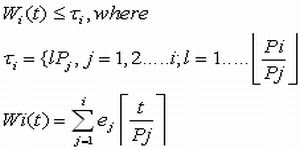Rate Monotonic Scheduling (RM)

Rate Monotonic Scheduling is the optimal static priority algorithm. Shortly it is referred as RM or RMA or RMS. To solve for the RM schedule, the following are the assumptions.

Assumptions:

• All the tasks are assumed to be periodic
• The relative deadline of the tasks are equal to its period.
• No tasks has a non pre-emptible section.
• The cost of preemption is negligible.

RMA

1. Priorities are assigned based on the periods of the task.
1. Lower the period, higher the priority
2. As rate is the inverse of the period, higher the rate, higher the priority
2. It is a static priority algorithm (priorities are assigned to tasks during their compilation time)

Schedulability Test for RMA

To find the schedulability Test of RM algorithm

• the sufficient condition for schedulability test for RM is
• The necessary and sufficient condition for testing the schedulability test is1.2.